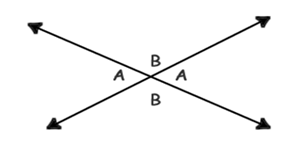# Mensuration

Mensuration is a branch of mathematics, which deals with the study of geometric shapes including their area, volume and other measurements.

## Lines and Angles

A line is defined as a long thin mark whereas an angle is the space between two straight intersecting lines. It is usually measured in terms of the amount of rotation.

LINES AND ANGLES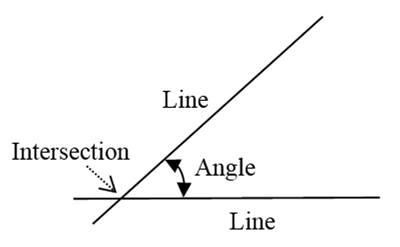An angular measurement is made in degrees and denoted as ‘o’. For example, 45o. Various degrees of a circle is given in the following figure.

30o REFERENCE ANGLES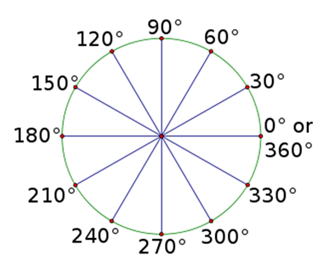In some disciplines, angles are measure in radians. It is numerically equal to the length of a corresponding arc of a unit circle. One radian is equal to 57.3o and is abbreviated to rads. One radian is the angle subtended at the circle center when the arc-length is equal in length to the radius.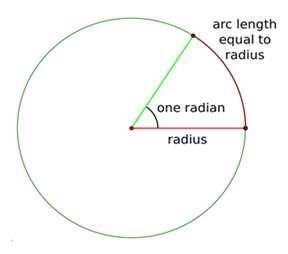Generally radians are quoted as follows.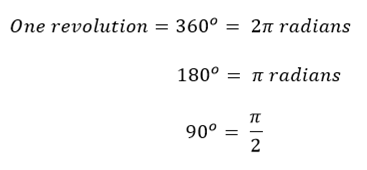The formula for converting degrees to radians is to multiply the number by Π/180°. The value of Π = 3.142.

Example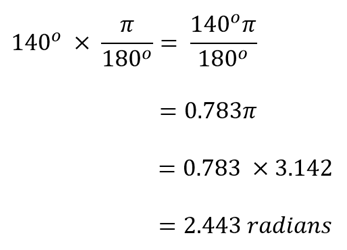The formula for converting degrees to radians is to multiply the number by 180°/Π. The value of Π = 3.142.

Example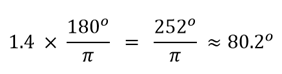### Types of Angles

Right angle: This angle measures exactly 90o and is indicated by a small square at the intersection point.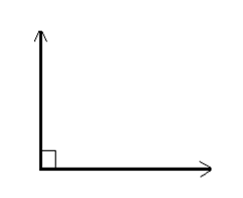Acute angle: This angle measures less than 90o.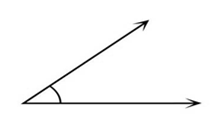Obtuse angle: This angle measures more than 90o but less than 180o.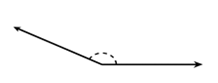Straight angle: This angle exactly 180o and looks like a straight line.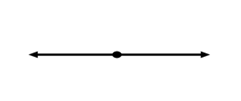Reflex angle: This angle measures more than 180o but less than 360o.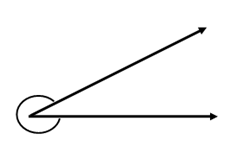Adjacent angle: This angle has a common vertex and a common side, which means that the vertical point and the side are shared by the two angles without overlapping.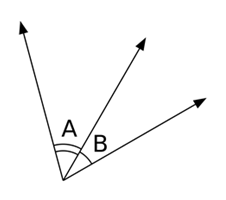Complimentary angles: The two angles whose measure add to 90o and form a right angle together are called complementary angles.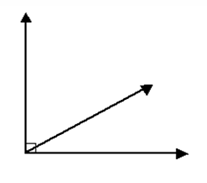Supplementary angles: The two angles whose measures add to 180o are called supplementary angles.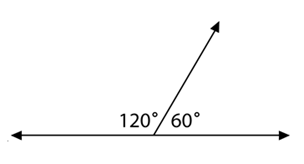Vertical angles: These angles are opposite to each other when two lines cross by sharing the same vertex.